# KVPY-SX 2018 Physics Paper with Solutions

KVPY (Kishore Vaigyanik Protsahan Yojana) is a scholarship examination conducted for students willing to pursue a degree in basic sciences like Mathematics, Chemistry, Physics etc. The objective of the test is to encourage students to make a career in science research. The KVPY SX 2018 Physics paper includes questions from topics like Heat and thermodynamics, Rotational motion, Fluid mechanics, Electrostatics, etc. The subject experts have solved these problems using the simplest approach.

### KVPY SX 2018 - Physics

Question 1: A table has a heavy circular top of radius 1 m and mass 20 kg, placed on four light (considered massless) legs placed symmetrically on its circumference. The maximum mass that can be kept anywhere on the table without toppling it is close to

1. a. 20 kg
2. b. 34 kg
3. c. 47 kg
4. d. 59 kg

Solution:

Given: Radius of circular table = 1 m

Mass of the table = 20 kg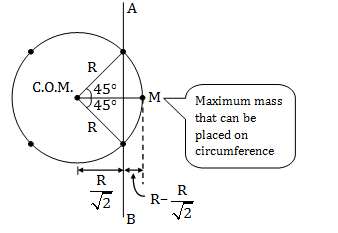$20g\left [ \frac{R}{\sqrt{2}} \right ]=MgR\left [ 1-\frac{1}{\sqrt{2}} \right ]$

$M\left [ \sqrt{2}-1 \right ]=20$

M = 48.28 kg

Hence, correct option is (c).

Question 2: Air (density ρ) is being blown off a soap film (surface tension T) by a pipe of radius R with its opening right next to the film. The film is deformed and a bubble detaches from the film when the shape of the deformed surface is a hemisphere. Given that the dynamic pressure on the film due to the air blown at speed v is (1/2) ρv2, the speed at which the bubble is formed is

1. a. $\sqrt{\frac{T}{\rho R}}$
2. b. $\sqrt{\frac{2T}{\rho R}}$
3. c. $\sqrt{\frac{4T}{\rho R}}$
4. d. $\sqrt{\frac{8T}{\rho R}}$

Solution:

The rate of formation of hemi-spherical bubble is same as air-entering speed inside the bubble.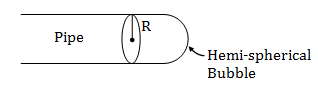For bubble formation:

Excess-pressure [ΔP] = 4T/R

Where, T = Tension in bubble, R = Radius of hemispherical bubble

This excess pressure provided by Dynamic-pressure on the film.

$\frac{1}{2}\rho v^{2}=\frac{4T}{R}$

$v=\sqrt{\frac{8T}{\rho R}}$

Hence, correct option is (d).

Question 3: For an ideal gas the internal energy is given by U = 5PV/2 + C, where C is a constant. The equation of the adiabats in the PV plane will be:

1. a. P5V7 = constant
2. b. P7V5 = constant
3. c. P3V5 = constant
4. d. P5V2 = constant

Solution:

The equation of adiabatic process in PV plane is:

PV γ = Constant (C) (P = Pressure, V=Volume, γ=ratio of specific heat)

Given: U = (5PV/2) + C

For an ideal gas:

PV = nRT

Where, R= Universal gas constant, T=Temperature, n = Number of moles

U =(5/2) nRT + C

Change in internal energy:

ΔU = (5/2)nR ΔT …(1)

We know:

ΔU =nf R ΔT/2 …(2)

From eq.(1) & (2):

Degree of freedom, f = 5

Now,

$\gamma =1+\frac{2}{f}=1+\frac{2}{5}=\frac{7}{5}$

So,

PV 7/5 = C

⇒ [PV 7/5]5 = C5

⇒ P5V7 = Constant

Hence, correct option is (a).

Question 4: An ideal gas undergoes a change in its state from the initial state I to the final state F via two possible paths as shown. Then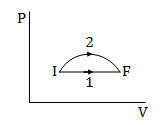1. a. there is no change in internal energy along path 1
2. b. heat is not absorbed by the gas in both paths
3. c. the temperature of the gas first increases and then decreases for path 2
4. d. work done by the gas is larger in path 1

Solution:

•Internal-energy is a state function. Hence, there is a change in U along-path 1.

•Area under the curve represents work-done on PV axis. Hence, option (D) is wrong.

• For an ideal gas

PV = nRT

$T=\left [ \frac{PV}{nR} \right ]$

•Firstly PV increases in path 2 hence temperature increases and then PV decreases in path 2 then temperature decreases.

Hence, option (C) is correct.

Question 5: A thermally insulated rigid container of one litre volume contains a diatomic ideal gas at room temperature. A small paddle installed inside the container is rotated from the outside such that the pressure rises by 105 Pa. The change in internal energy is close to

1. a. 0 J
2. b. 67 J
3. c. 150 J
4. d. 250 J

Solution: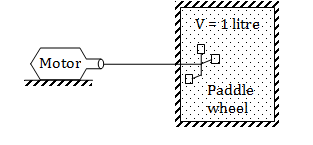dQ = 0

dU = dW

ΔU = Work done in adiabatic process

$\Delta U=\frac{P_{1}V_{1}-P_{2}V_{2}}{\gamma -1}$

$\Delta U=-\frac{\left [P_{2}-P_{1} \right ]V}{{\gamma -1}}$

$\Delta U=-\frac{\Delta PV}{{\gamma -1}}$ {given: V=1litre, Pressure rises= 105Pa}

$=\frac{10^{5}\times 10^{-3}m^{3}}{\frac{7}{5}-1}$ (for diatomic gas γ = 7/5)

Hence, correct option is (d).

Question 6: In Young’s .double-slit experiment the amplitudes of the two wave’s incident on the two slits are A and 2A. If I0 is the maximum intensity, then the intensity at a spot on the screen where the phase difference between the two interfering waves is φ.

1. a. I0cos2(φ/2)
2. b. (I0 /3)sin2(φ/ 2)
3. c. (I0 /9) (5 + 4cos(φ))
4. d. (I0 /9) (5 + 8cos(φ))

Solution: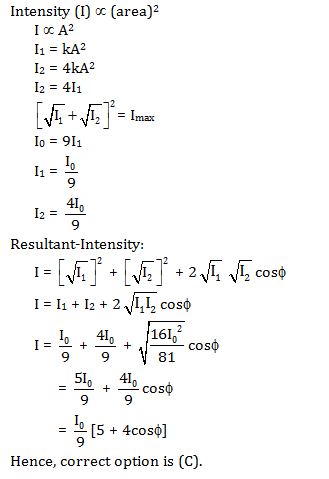Question 7: Figures below show water flowing through a horizontal pipe from left to right. Note that the pipe in the middle is narrower. Choose the most appropriate depiction of water levels in the vertical pipes.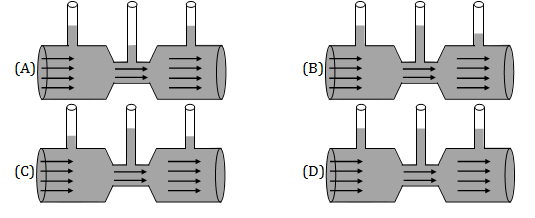Solution:

Recall Bernoulli’s equation:

$\frac{P}{\rho g}+\frac{V^{2}}{2g}+Z=constant$

Where, $\frac{P}{\rho g}$= pressure head,

$\frac{V^{2}}{2 g}$ = kinetic head

Z = datum/reference level

For flow to be possible:

A1V1 = A2V2 (From the equation of continuity)

$V\propto \frac{1}{A}$

As area decreases velocity increases at narrow-section.

•As velocity increases; pressure decreases.

Hence, the correct option is (a).

Question 8: A plank is moving in a horizontal direction with a constant acceleration. A uniform rough cubical block of side ℓ rests on the plank, and is at rest relative to the plank.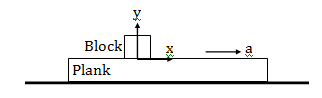Let the center of mass of the block be at (0, ℓ/2) at a given instant. If a = g/10, then the normal reaction exerted by the plank on the block at that instant acts at

1. a. (0, 0)
2. b. (–ℓ/20, 0)
3. c. (–ℓ/10, 0)
4. d. (ℓ/10, 0)

Solution:

Given: a=g/10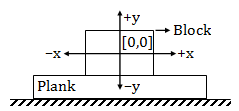Observing block with respect to plank: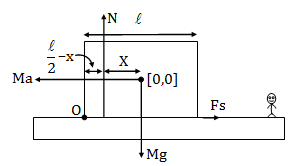For rotational equilibrium:

$\Sigma \tau _{0}=0$

$Mg\frac{l}{2}=\frac{Mg}{10}\frac{l}{2}+Mg\left [ \frac{l}{2}-x \right ]$

X=l/20

The normal reaction act at:

$\left [ -\frac{l}{20},0 \right ]$

Hence, correct option is (b).

Question 9: Using the Heisenberg uncertainty principle, arrange the following particles in the order of increasing lowest energy possible.

(I) an electron in H2 molecule

(II) a H atom in a H2 molecule

(III) a proton in the carbon nucleus

(IV) a H2 molecule within a nanotube

1. a. (I) < (III) < (II) < (IV)
2. b. (IV) < (II) < (I) < (III)
3. c. (II) < (IV) < (III) < (I)
4. d. (IV) < (I) < (II) < (III)

Solution:

Heisenberg uncertainty principle

(Δx)( ΔP) ≥ h/4 π

E =p2/2m (The relation between kinetic energy and momentum)

• For option (III): Uncertainty in position (Δx) is quite small hence (ΔP) is quite large hence increase in lowest energy possible (ΔE) is maximum for this condition.

• For option (IV): Uncertainty of position of H2 molecule in a nanotube is more hence uncertainty in energy (ΔE) is quite small.

• Similarly for option (I) & (II):

Uncertainty of position (Δx) of the electron (e–) in H2 molecule

<Uncertainty of position (Δx) of H atom in H2 molecule

Hence,

Uncertainty of momentum/increase in the lowest energy of an electron in H2 molecule

>Uncertainty of momentum/increase in lowest energy of H atom is H2 molecule.

Hence, using the above concept

IV < II < I < III

Hence, option (b) is the best possible answer.

Question 10: The current is flowing along the path abcd of a cube (shown to the left) produces a magnetic field at the centre of a cube of magnitude B. Dashed line depicts the non-conducting part of the cube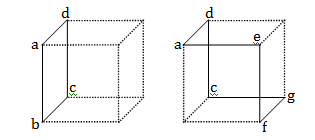Consider a cubical shape shown to the right which is identical in size and shape to the left. If the same current now flows in along the path daefgcd, then the magnitude of the magnetic field at the centre will be:

1. a. zero
2. b. $\sqrt{2}B$
3. c. $\sqrt{3}B$
4. d. B

Solution: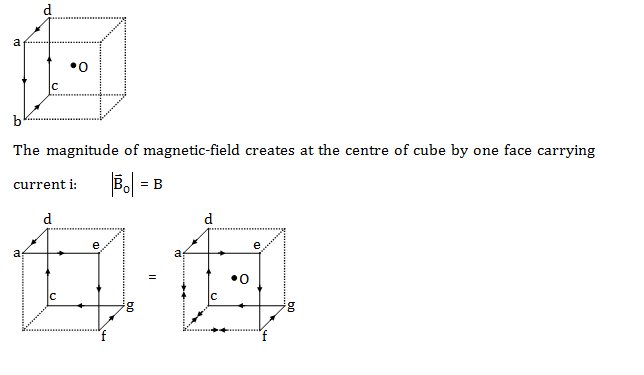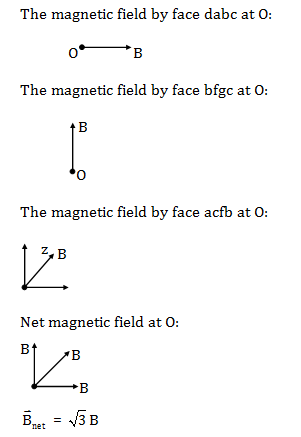Hence, the correct option is (C).

Question 11: A thin metallic disc is rotating with constant angular velocity about a vertical axis that is perpendicular to its plane and passes through its centre. The rotation causes the free electrons in the disc to redistribute. Assume that there is no external electric or magnetic field. Then

1. a. a point on the rim of the disc is at a higher potential than the centre.
2. b. a point on the rim of the disc is at a lower potential than the centre.
3. c. a point on the rim of the disc is at the same potential as the centre.
4. d. the potential in the material has an extremum between centre and the rim.

Solution: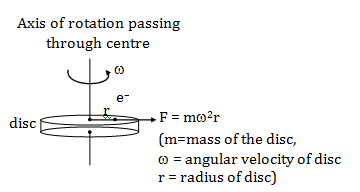•Due to centrifugal force electrons have a tendency to go towards the circumference.

•As electron gather on circumference it will generate negative potential on circumference and deficiency of electron at the centre it will generate higher potential at the centre.

Hence, the correct option is (b).

Question 12: One mole of a monatomic gas and one mole of a diatomic gas are initially in the same state. Both gases are expanded isothermally and then adiabatically such that they acquire the same final state. Choose the correct statement.

1. a. Work done by diatomic gas is more than that by monatomic gas
2. b. Work done by monatomic gas is more than that by diatomic gas
3. c. Work done by both the gases are equal
4. d. Change in internal energies of both the gases are equal

Solution: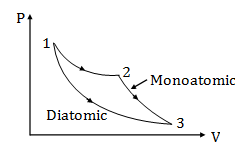•Recall the concept of slope in isothermal and adiabatic process.

$\left [ \frac{dP}{dV}=-\frac{\gamma P}{V} \right ]$

$\left [\gamma _{diatomic}=\frac{7}{5};\gamma _{monoatomic}=\frac{3}{2} \right ]$

•Area under the curve of the diatomic process is less, hence work done is less.

Hence, the correct option is (b).

Question 13: An ideal gas is made to undergo the cyclic process shown in the figure below. Let ΔW depict the work done, ΔU be the change in internal energy of the gas and Q be the heat added to the gas. Sign of each of these three quantities for the whole cycle will be (0 refers to no change)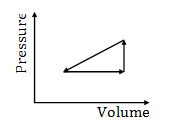1. a. –, 0, –
2. b. +, 0, +
3. c. 0, 0, 0
4. d. +, +, +

Solution: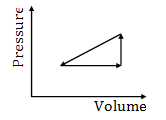Cycle is anti-clockwise. Hence

Work done W = –ive

For a cycle initial & final state are same. Hence

Change in internal energy ΔU = 0

For a cycle:

ΣQ = ΣW

Change in heat ΔQ = –ive

Hence, the correct option is (A).

Question 14: Two balls of mass M and 2M are thrown horizontally with the same initial velocity v0 from top of a tall tower and experience a drag force of –kv(k > 0), where v is the instantaneous velocity. Then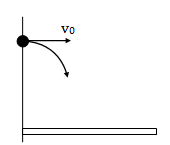1. a. The heavier ball will hit the ground further away than the lighter ball
2. b. The heavier ball will hit the ground closer than the lighter ball
3. c. Both balls will hit the ground at the same point
4. d. Both balls will hit the ground at the same time

Solution: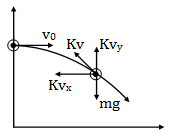Both heavier and lighter ball has ux = v0

Now retardation in x-direction

$a_{x}=\frac{F_{x}}{m}$ (Fx = force in x-direction, m=mass of the ball)

$a_{x}=\frac{KV_{x}}{m}$

$a_{x}\propto \frac{1}{m}$

So, heavier block will hit the ground further away than the lighter ball.

Hence, correct option is (a).

Question 15: Consider a glass cube slab of dielectric bound by the planes x = 0, x = a; y = 0, y = b; z = 0, z = c; with b > a > c. The slab is placed in air and has a refractive index of n. The minimum value for n such that all rays entering the dielectric at y = 0 reach y = b is

1. a. 1
2. b. $\sqrt{2}$
3. c. $\sqrt{3}$
4. d. 2

Solution: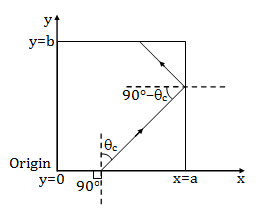Recall TIR [Total internal reflection]

$\theta \geq \theta _{c}$

$90-\theta_{c} \geq \theta _{c}$

$\theta_{c} \leq 45^{0}$

$sin\theta_{c} \leq sin 45^{0}$

$\frac{1}{n} \leq \frac{1}{\sqrt{2}}$

$n\geq \sqrt{2}$

Minimum value of refractive index n should be $\sqrt{2}$.

Hence, correct option is (b).

Question 16: The graph shows the log of activity (log R) of a radioactive material as a function of time t in minutes.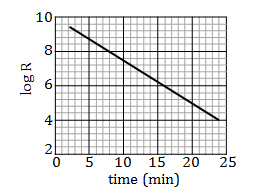The half-life (in minutes) for the decay is closest to

1. a. 2.1
2. b. 3.0
3. c. 3.9
4. d. 4.4

Solution: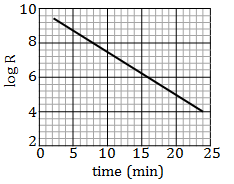R = Roe– λt

Taking log:

log R = log Ro – λt [y = mx + c]

Graph of log R vs time is straight line and its slope is λ. [Decay-constant]

$-\lambda =\left [ \frac{8-6}{16-8} \right ]$

$\lambda =\frac{1}{4}$

$T_{1/2}=ln2/\lambda$

= 0.693/(1/4)

= 2.772 minute

Closest value is 3 minutes.

Hence, the correct option is (b).

Question 17: The magnetic field is uniform for y > 0 and points into the plane. The magnetic field is uniform and points out of the plane for y < 0. A proton denoted by filled circle leaves y = 0 in the y-direction with some speed as shown below.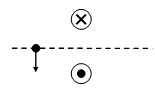Which of the following best denotes the trajectory of the proton.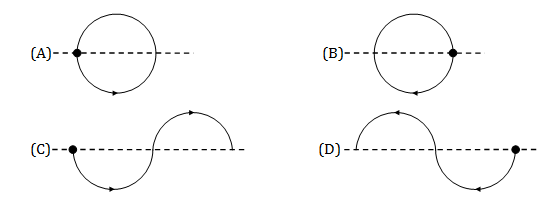Solution:

Concept: Charge entering into a uniform magnetic field.

When charge (q) enter into a uniform magnetic field (B) with velocity (V) then force on the charge.

$F=q\left [ \vec{V}\times \vec{B} \right ]$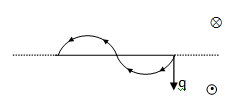Correct option is (d).

Question 18: The Hitomi satellite recently observed the Lyman alpha emission line (n = 2 to n = 1) of Hydrogen-like iron ion (atomic number of iron is 26) from the Perseus galaxy cluster. The wavelength of the line is closest to

1. a. 2Å
2. b. 1Å
3. c. 50Å
4. d. 10Å

Solution:

Line of emission OR emission-spectrum depends on the energy radiated by photon.

Energy of a photon

E = hc / λ = 12400 Å / λ

$E=13.6Z^{2}\left [ \frac{1}{n_{1}^{2}}-\frac{1}{n_{2}^{2}} \right ]$----------(2)

Z = atomic number

From eq.(1) & (2)

$\frac{12400}{\lambda }=13.6^{2}\left [ \frac{1}{1}-\frac{1}{4} \right ]$

λ = 12400 Å X 4 / 13.6 X 676 X 3

λ = 1.79 ≈ 2 Å

Hence, correct option is (a).

Question 19: Assume that the drag force on a football depends only on the density of the air, the velocity of the ball and the cross-sectional area of the ball. Balls of different sizes but the same density are dropped in an air column. The terminal velocity reached by balls of masses 250 g and 125 g are in the ratio:

1. a. 21/6
2. b. 21/3
3. c. 21/2
4. d. 22/3

Solution:

Given, drag force FD depends on air density ρ, the velocity of ball v and area of cross-section of ball A.

FD ∝ ρxvyAz

Writing the dimensional-formula for r, v, A & FD:

[FD] = [M1L1T–2]

[ρ] = [M1L–3]

[v] = [L1T–1]

[A] = [L2]

[M1L1T–2] ∝ [M1L–3]x[L1T–1]y[L2]z

[M1L1T–2] ∝ [M]x[L] –3x+y+2z[T] –y

On comparing:

x = 1

y = 2

z =(3x-y)/2 = 1

FD∝ρ v2A

FD = Kρv2A

Where K is proportionality constant which has a value of 1/2.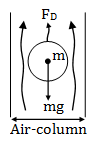At terminal velocity, ball must be in Equilibrium. So

FD = mg

KρAv2 = mg

As density is the same: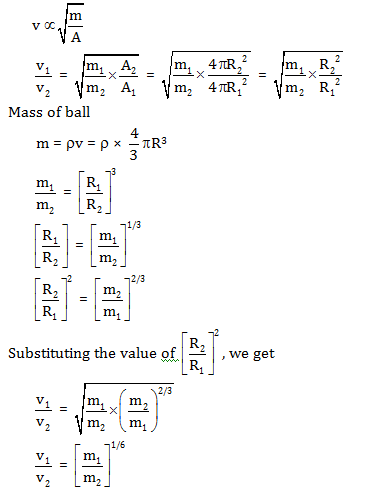= $\left [ \frac{250 g}{125g} \right ]^{1/6}$

= 2 1/6

Hence, the correct option is (a).

Question 20: An electrostatic field line leaves at an angle α from point charge q1 and connects with point charge –q2 at an angle β (q1 and q2 are positive) (see figure below). If q2 = q1 and α = 30°, then

1. a. 0° < β < 30°
2. b. β = 30°
3. c. 30° < β< 60°
4. d. 60° < β <90°

Solution: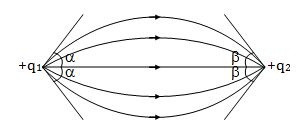Now EF field lines emerges from q1 and terminate at –q2.

Number of electric-field lines ∝ Magnitude of charge ∝ Electric flux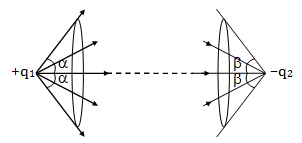The relation between plane angle and solid angle.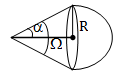$\Omega =2\pi [1-cos\alpha ]$

Electric flux coming out of q1= Electric flux entering at q2

$\frac{q_{1}}{4\pi \varepsilon _{0}}2\pi [1-cos\alpha ]=\frac{q_{2}}{4\pi \varepsilon _{0}}2\pi [1-cos\beta ]$

$\frac{q_{1}}{q_{2}} =\frac{[1-cos\beta ]}{[1-cos\alpha ]}$

$\frac{q_{1}}{q_{2}} =\frac{2sin^{2}\frac{\beta }{2}}{2sin^{2}\frac{\alpha }{2}}$

$\left [ \frac{2}{3} \right ]\times sin^{2}15^{0}=sin^{2}\frac{\beta }{2}$

$\beta =24.40^{0}$

Hence, correct option is (a).

Question 21: In steady state heat conduction, the equations that determine the heat current $\vec{J}(\vec{r})$ [heat flowing per unit time per unit area] and temperature $T(\vec{r})$ in space are exactly the same as those governing the electric field $\vec{E}(\vec{r})$ and electrostatic potential $V(\vec{r})$ with the equivalence given in the Table below:

 Heat flow Electrostatics $T(\vec{r})$ $\vec{J}(\vec{r})$ $V(\vec{r})$ $\vec{E}(\vec{r})$

We exploit this equivalence to predict the rate Q of total heat flowing by conduction from the surface of spheres of varying radii, all maintained at the same temperature. If Q ∝Rn, (here R is the radius), then the value of n is

1. a. 2
2. b. 1
3. c. –1
4. d. –2

Solution:

 Heat flow Electrostatics $T(\vec{r})$ $\vec{J}(\vec{r})$ $V(\vec{r})$ $\vec{E}(\vec{r})$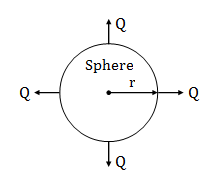Rate of heat-flowing by conduction from the surface of the sphere:

Q= –KA (dT/dr)

Q = –K4 πr2(dT/dr)

$\frac{Q}{K(4\pi r^{2})}dr=-dt$ …(1)

•As temperature is co-related with Electric-potential.

We know for reference:

r = ∞ v(∞) = 0

Similarly from given analogy

r = ∞ ; T(∞) = 0

r = r ; V(r) = V

r = r ; T(r) = T

Applying limits to equation (1):

$\int_{R}^{\infty} \frac{\dot{Q}}{4 \pi \mathrm{Kr}^{2}} dr=\int_{\mathrm{T}}^{0}-\mathrm{dT}$

$\frac{\overline{\mathrm{Q}}}{4 \pi \mathrm{K}}\left[\frac{-1}{\mathrm{r}}\right]_{R}^{\infty}=[-\mathrm{T}]_{\mathrm{R}}^{0}$

$\frac{\mathrm{Q}}{4 \pi \mathrm{K}}\left[\frac{1}{\mathrm{R}}\right]=[\mathrm{T}]$

Q= 4 πKRT

Q ∝Rn (given)

n = 1

Hence, correct option is (b).

Question 22: An arrangement of spring, strings, pulley and masses is shown in the figure. The pulley and the strings are massless and M > m. The spring is light with spring constant k. If the string connecting m to the ground is detached, then immediately after detachment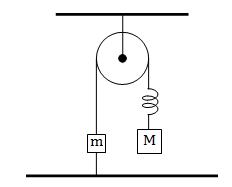1. a. the magnitude of the acceleration of m is zero and that of M is g
2. b. the magnitude of the acceleration of m is (M – m)g/m and that of M is zero.
3. c. the accelerations of both masses are the same
4. d. the elongation in the spring is (M – m)g/k

Solution:

The system is in equilibrium now,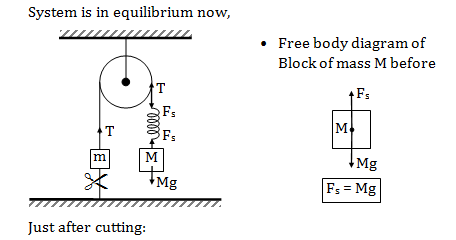• Spring is non-impulse force hence it will not suddenly wipe out as you cut the string

For block M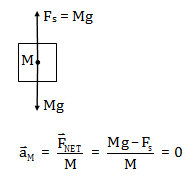As tension in the string is a self-adjusting force. As Fs is there, T will be there in the string.

For block m: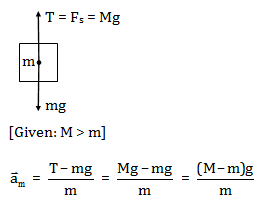Hence, the correct option is (b).

Question 23: The potential due to an electrostatic charge distribution is

$V(r)=\frac{qe^{-\alpha r}}{4\pi \varepsilon _{0}r}$

Where α is positive. The net charge within a sphere centred at the origin and of radius 1/ α is

1. a. 2q/e
2. b. (1 – 1/e)q
3. c. q/e
4. d. (1 + 1/e)q

Solution: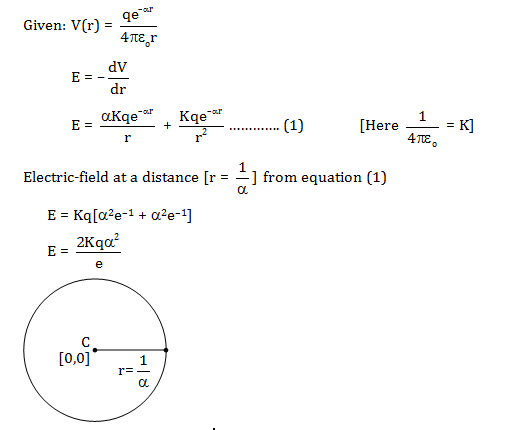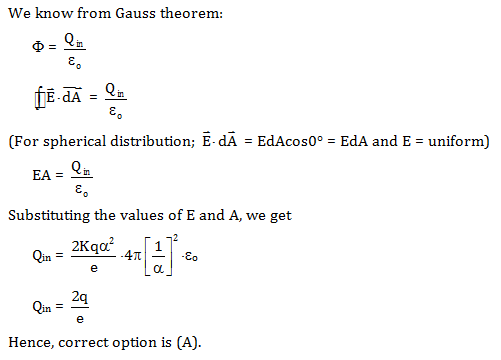Question 24: A wheel of radius R is trapped in a mud pit and spinning. As the wheel is spinning, it splashes mud blobs with initial speed u from various points on its circumference. The maximum height from the centre of the wheel, to which a mud blob can reach is

1. a. $\frac{u^{2}}{2g}$
2. b. $\frac{u^{2}}{2g}+\frac{gR^{2}}{2u^{2}}$
3. c. $\frac{u^{2}}{g}$
4. d. $R+\frac{u^{2}}{2g}$

Solution:

Let a mud blob is detached from the circumference of the wheel with initial speed u at an angle θ as shown below.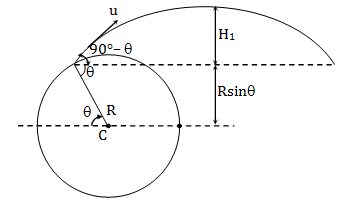Estimating the problem for a particular angle θ.

H = H1 + R sin θ

H1 = Hmax for projectile leaving at an angle (90- θ)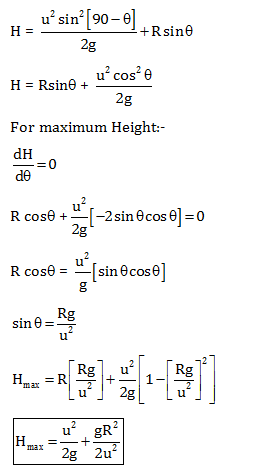Hence, option (b) is correct.

Question 25: Two rods of copper and iron with the same cross sectional area are joined at S and a steady current I flows through the rods as shown in the figure.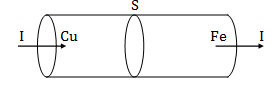Choose the most appropriate representation of charges accumulated near the junction S.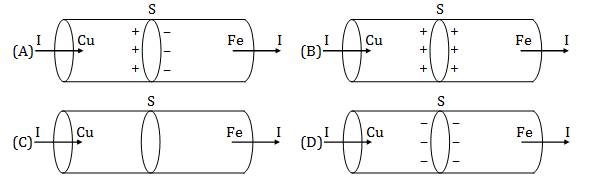Solution: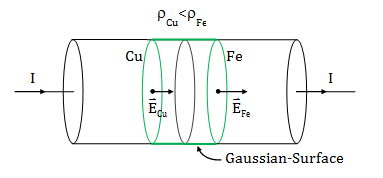From the definition of current density i.e. current per unit area is given by

J = I/S = same

Where, J = current density, I = current, S = cross-sectional area

The relation between current density and thermal conductivity is given by

$\vec{J}=\sigma \vec{E}=\frac{\vec{E}}{\rho }$

Where, σ = conductivity, ρ = resistivity, $\vec{E}$ = electric field intensity

$\vec{E}=\rho \vec{J}$

$\left |\vec{E}_{Fe} \right |> \left | \vec{E}_{Cu} \right |$

Net flux through Gaussian-surface:

$\phi =E_{Fe}S-E_{Cu}S$ $[\phi > 0 \: as\: \left |\vec{E}_{Fe} \right |> \left | \vec{E}_{Cu} \right |]$

[Flux through curved surface is zero as $\vec{E}\perp \vec{A}$ ]

$\phi =\frac{\Sigma Q_{in}}{\varepsilon _{0}}=(+ive)$

ΣQin = +ive [Accumulated charge on x-section should be positive]

Hence, correct option is (b).

Question 26: Graphs below show the entropy vs energy (U) of two systems I and II at constant volume. The initial energies of the systems are indicated by UI,i and UII,i, respectively. Graphs are drawn to the same scale. The systems are then brought into thermal contact with each other. Assume that at all times the combined energy of the two systems remains constant. Choose the most appropriate option indicating the energies of the two systems and the total entropy after they achieve the equilibrium.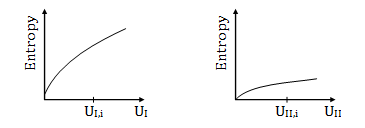1. a. UI increases and UII decreases and the total entropy remains the same
2. b. UI decreases and UII increases and the total entropy remains the same
3. c. UI increases and UII decreases and the total entropy increases
4. d. UI decreases and UII increases and the total entropy increases

Solution: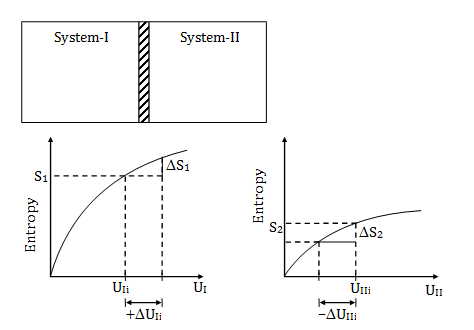Utotal = UIi + UIIi = constant

[ΔS]system = [ΔS]1 + [ΔS]2

$[\Delta S]_{system}|_{initial}=S_{1}+S_{2}$

As total energy of the system remains the same

|ΔUIi| = | ΔUIii|

|ΔS|I> |ΔS|II (from the graph)

Checking option (C); now entropy after thermal equilibrium:

By second law of thermodynamics, the entropy of a system either increases or remains constant (only in reversible quasi-static processes)

[ΔS]system = [S1 +ΔS1] + [S2 – ΔS2]

As, ΔS1>ΔS2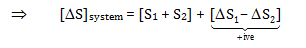Hence, the correct option is (c).

Question 27: The image of an object O due to reflection from the surface of a lake is elongated due to the ripples on the water surface caused by a light breeze. This is because the ripples act as tilted mirrors as shown. Consider the case where O and the observer E are at the same height above the surface of the lake. If the maximum angle that the ripples make with the horizontal is α, the angular extent δ of the image will be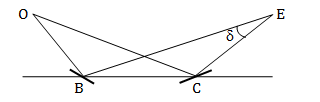1. a. α/2
2. b. α
3. c. 2α
4. d. 4 α

Solution: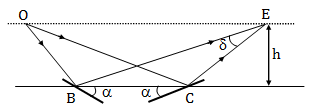We can consider ripples as plane mirrors reflecting light to form an image with angular extent δ.

It is given O and E are at the same level, so OEBC is a trapezium with OE||BC.

Now, different angles of trapezium OEBC are shown below.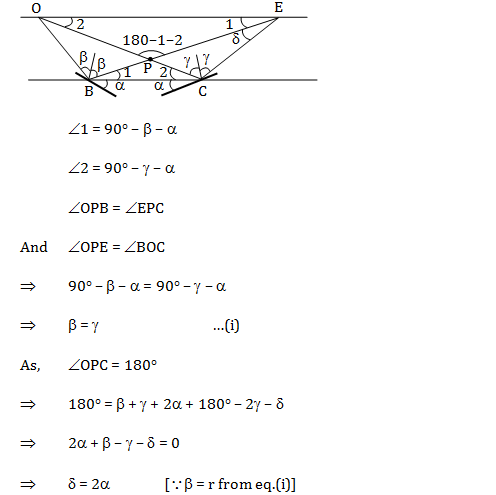Question 28: A spiral galaxy can be approximated as an infinitesimally thin disk of a uniform surface mass density (mass per unit area) located at z = 0. Two stars A and B start from rest from heights 2z0 and z0 (z0<< radial extent of the disk), respectively, and fall towards the disk, cross over to the other side, and execute periodic oscillations. The ratio of time periods of A and B is

1. a. 2–1/2
2. b. 2
3. c. 1
4. d. 2 1/2

Solution: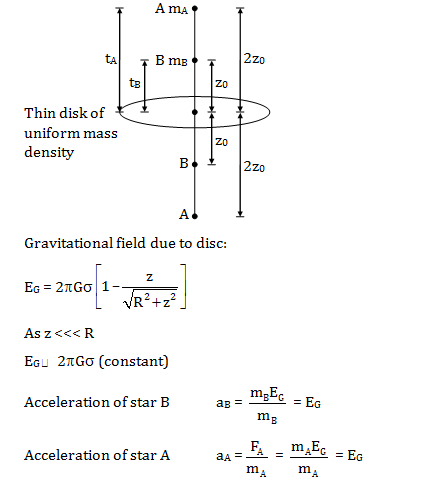For Star-A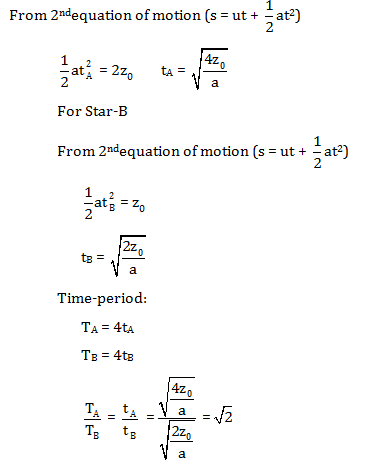Hence, the correct option is (d).

Question 29: Two mutually perpendicular infinitely long straight conductors carrying uniformly distributed charges of linear densities λ1 and λ2 are positioned at a distance r from each other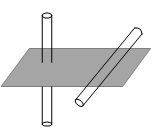The force between the conductors depends on r as

1. a. l/r
2. b. l/r2
3. c. r
4. d. r0

Solution: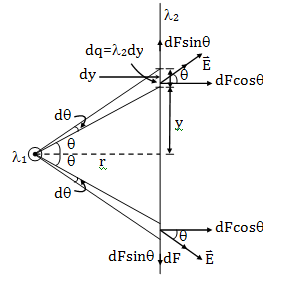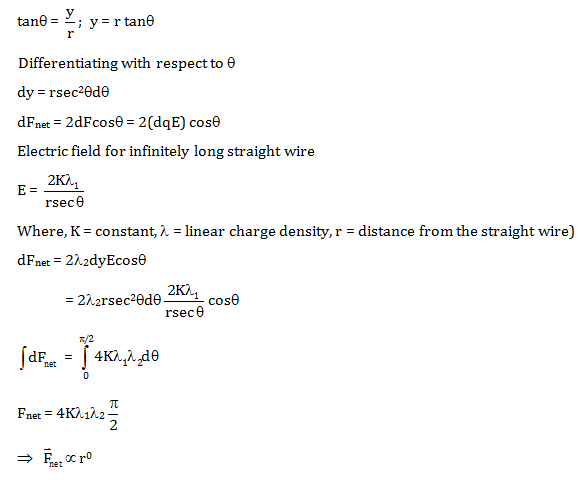Hence, the correct option is (d).

Question 30: The graph below shows the variation of a force (F) with time (t) on a body which is moving in a straight line. Dependence of force on time is F ∝tn. Initially body is at rest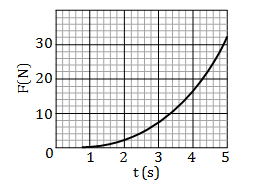If the speed of the object is 2 m/s at 3 s, the speed at 4 s will be approximately (in m/s)

1. a. 2.5
2. b. 6.5
3. c. 7.8
4. d. 3.1

Solution:

Given:

Dependence of force on time:

F ∝ tn

F = Ktn …(a)

Where K is constant.

From graph:

t = 2 sec; F = 2N

2 = Kn …(1)

t = 4 sec; F = 16N

16 = Kn …(2)

Dividing eq.(2)/eq.(1)

n = 8

n = 3

Putting n value in eq.(a)

F = Kt3

We know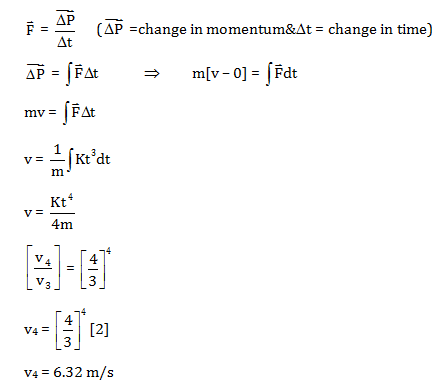Hence, correct option is (b).

### KVPY SX 2018 Physics Paper with Solutions